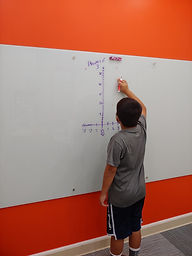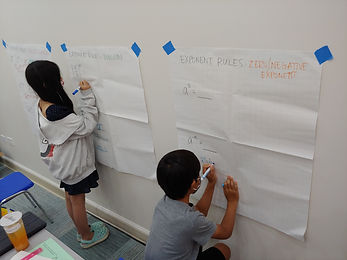Ms. Mari

Target 1​

Lesson Type:

Review

Algebra

:

Variable

Determine the unknown integer in an equation relating three whole numbers.

1:

Solve problems where there is one piece of information in the equation missing, applying understandings of operations.

8th

Vocabulary:

Activities:

Used algebraic methods to arrange the digits 1-9 around a triangular holiday tree so that each set of four numbers on each side summed to the same total.Home Exploration

Guiding Questions:Absent Students:

Jacob

Target 2

:

1:

Determine the exponential rules or laws that apply to multiplication and division.

2:

Use the product rule to multiply expressions with like bases (ax * ay = ax+y).

3:

Use the quotient rule to divide expression with like bases (ax ÷ ay = ax-y).

4:

Solve expressions using exponential rules and laws.

8th

Vocabulary:

PRODUCT OF POWERS, QUOTIENT OF POWERS, POWER OF A POWER, POWER OF A PRODUCT, POWER OF A QUOTIENT, ZERO EXPONENT, NEGATIVE EXPONENT

Activities:

- Used powers of 3 to determine rules for zero and negative exponents.

- Created a graph for y=3^x to observe and represent an exponential relationship.

- Explored and determined rules for multiplication and division with exponents.

- Organized the rules on a poster and created examples for each rule.Home Exploration

Guiding Questions:Target 3

:

Vocabulary:

Activities:Home Exploration